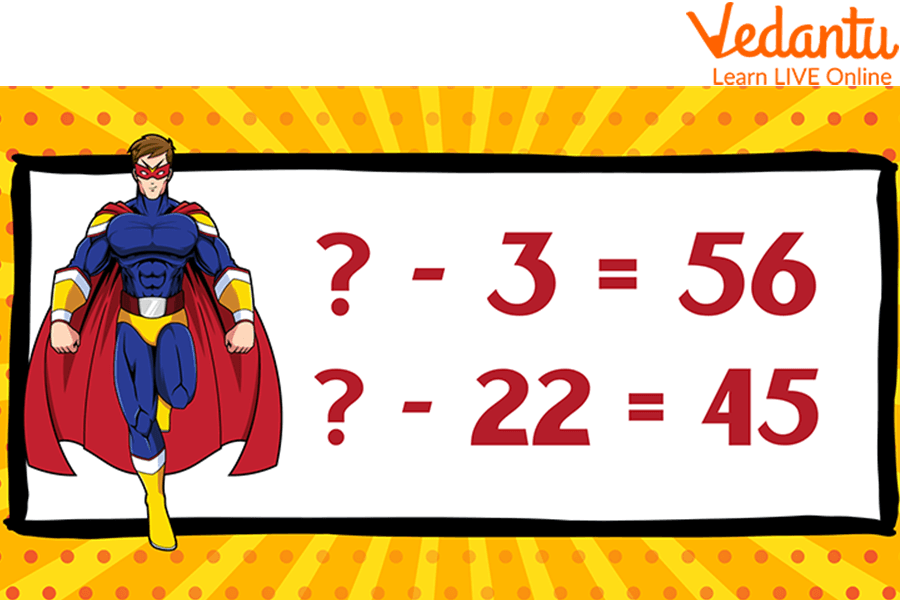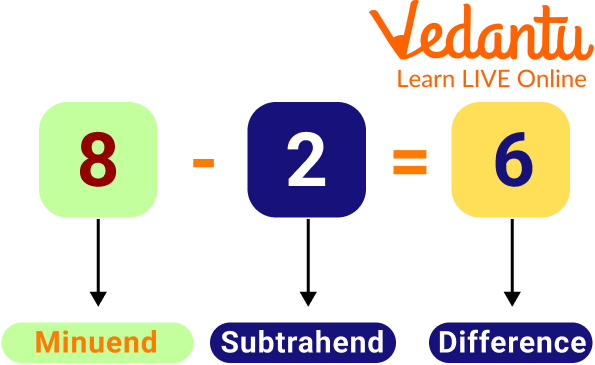Courses
Courses for Kids
Free study material
Free LIVE classes
More

# Subtraction Missing MinuendsLIVE
Join Vedantu’s FREE Mastercalss

## A Brief Explanation of Subtraction Missing Minuends

Missing numbers are the numbers that have been missed in a sequence or a series. We can find those missing numbers by identifying the pattern. Whenever there are blanks in a series of numbers, a few numbers have been missed, and we need to observe the pattern and fill in the blanks with suitable numbers. The minuend is the number from which you subtract another, while the subtrahend is the number you take away each step. Go through some minuend subtrahend difference worksheets to understand more clearly.Subtraction Missing Minuends

## What is Subtraction?

Subtraction is an operation used to find the difference between numbers. The group becomes smaller when you have a group of objects and take away a few objects from it. For example, you bought 9 cupcakes for your birthday party, and your friends ate 7 cupcakes. Now you are left with 2 cupcakes. This can be written as a subtraction expression: 9 - 7 = 2 and is read as "nine minus seven equals two". When we subtract 7 from 9 (9 - 7), we get 2. Here, we performed the subtraction operation on two numbers, 9 and 7, to get the difference of 2.

## Parts of Subtraction

When we subtract two numbers, we use some terms in the subtraction expression:

Minuend: The number from which the other number is subtracted.

Subtrahend: The number which is to be subtracted from the minuend.

Difference: The final result after subtracting the subtrahend from the minuend.

The subtraction formula is written as Minuend - Subtrahend = Difference,

i.e. Minuend = Difference + SubtrahendParts of subtraction

## Rules for Subtraction Minuend

Here is the rule for subtraction minuend, which is like this:

It is very easy to get the number given in the subtraction minuend, for which we will add both the given numbers together so that we will get the answer to the given question. We can verify the answer obtained by subtracting it from the given number to get back the same number as given in the question.Subtraction Missing Minuends

## Subtraction Minuend Related Solved Examples

Here are some examples related to the subtraction minuend, which are like this;

Example- What should be subtracted from 2256 so that we get 3516?

Thousands

Hundreds

Tens

Ones

—---

—-----

—----

—-

2

2

5

6

3

5

1

6

Ans:

 Thousands Hundreds Tens Ones 5 7 7 2 2 2 5 6 3 5 1 6

Thus the subtraction missing minuend is 5772.

Example- What should be subtracted from 5620 so that we get 7892?

 Thousands Hundreds Tens Ones —--- —--- —-- —-- 5 6 2 0 7 8 9 2

Ans:

 Thousands Hundreds Tens Ones 13 5 1 2 5 6 2 0 7 8 9 2

Thus, the subtraction missing minuend is 13512.

Example- What should be subtracted from 1562 so that we get 2556?

 Thousands Hundreds Tens Ones —-- —- —--- —--- 1 5 6 2 2 5 5 6

Ans:

 Thousands Hundreds Tens Ones 4 1 1 8 1 5 6 2 2 5 5 6

Thus the subtraction missing minuend is 4118.

## Minuend Subtrahend Difference Worksheets

Here are some practice problems related to the minuend, subtrahend and difference worksheets, which are like this;

Q1. What should be subtracted from 562 so that we get 300?

Ans. 262

Q2. What should be subtracted from 156 so that we get 56?

Ans. 100

Q3. What should be subtracted from 162 so that we get 96?

Ans. 66

Q4. What should be subtracted from 363 so that we get 126?

Ans. 237

Q5. What should be subtracted from 498 so that we get 121?

Ans. 377

## Summary

In this article, we have discussed the concept of subtraction missing minuends for kids. This article has provided a solution to the problem of learning subtraction minuend. It also discusses the rules of solving subtraction minuend problems. This section describes the importance of students knowing how to solve subtraction missing minuends problems.

In mathematics, the minuend is the sum of two or more numbers that are being subtracted from each other. The minuend is always greater than the subtrahend. In this article, we have talked about how to find the missing minuends in subtraction problems. We also discussed how to do it using a variety of methods.

Last updated date: 21st Sep 2023
Total views: 81k
Views today: 0.81k

## FAQs on Subtraction Missing Minuends

1. Where do we use subtraction?

Subtraction is used in our day-to-day life. For example, if we want to know how much money we spent on the items we bought, how much money is left with us, or if we want to calculate the time left to finish a task, we use subtraction.

2. What should be taken into account initially when subtracting integers?

Keep the first number first (known as the minuend). Second, switch from subtraction to addition in the procedure. Third, obtain the second number's opposing sign (the subtrahend). Finally, carry out the usual integer addition.

3. Are you adding and subtracting from left to right?

The order of operations is a collection of guidelines that mathematicians have adopted to specify which operation should be performed first. Here are the guidelines when an expression only contains the four fundamental operations: Divide and multiply from left to right. Subtract and add from left to right.